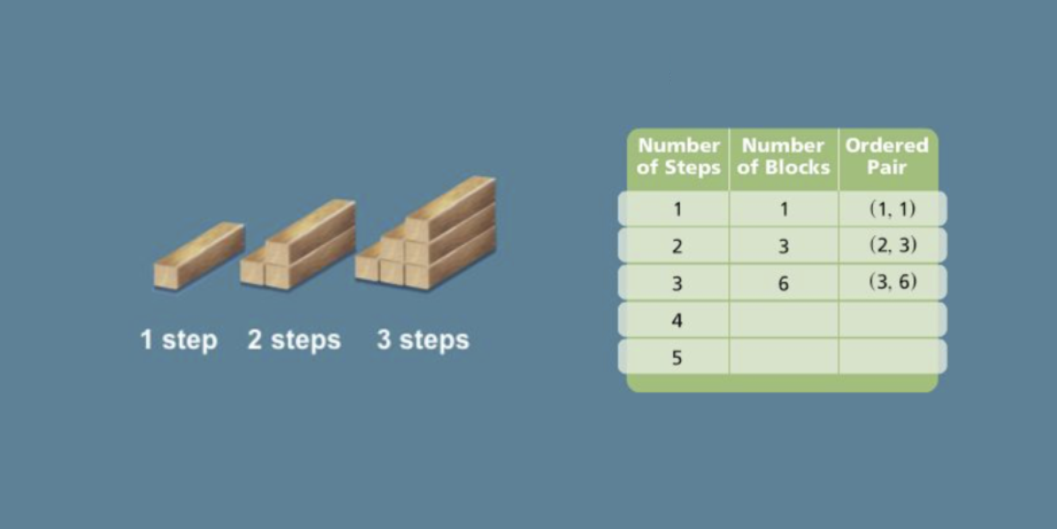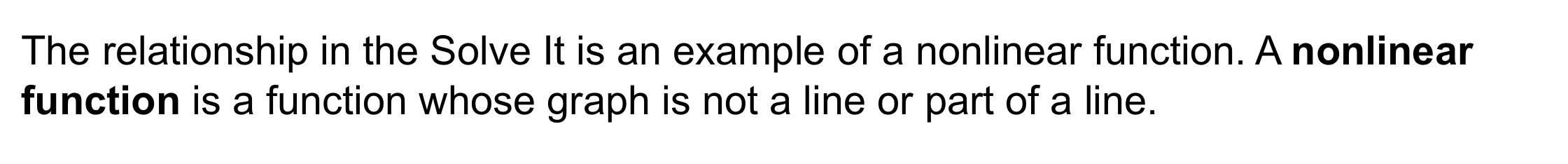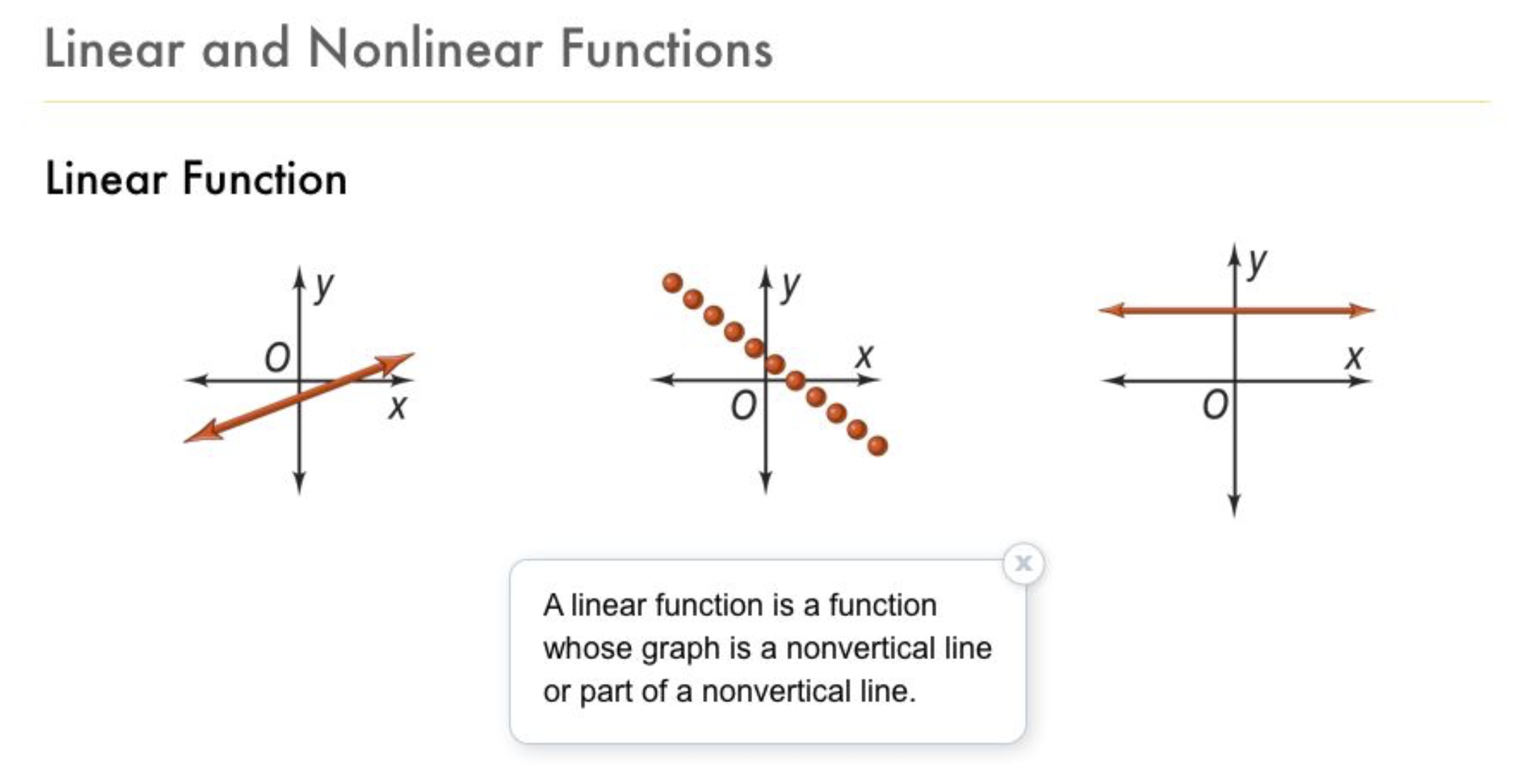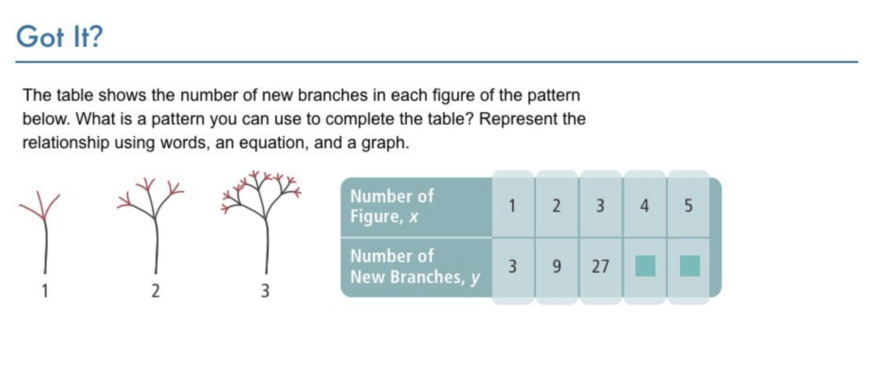Algebra 1 4-3 Complete Lesson: Patterns and Nonlinear Functions
starstarstarstarstarstarstarstarstarstar
4.5 (1 rating)
by Matthew Richardson
| 36 Questions
Note from the author:
A complete formative lesson with embedded slideshow, mini lecture screencasts, checks for understanding, practice items, mixed review, and reflection. I create these assignments to supplement each lesson of Pearson's Common Core Edition Algebra 1, Algebra 2, and Geometry courses. See also mathquest.net and twitter.com/mathquestEDU.Solve It! The table below shows the relationship between the number of steps in the staircase below and the number of blocks needed to build the staircase.1
2
3
4
1
10
Solve It! How many blocks are required to build 4 steps? Enter only a number.
2
1
Solve It! What is the ordered pair associated with 4 steps?
(4, 13)
(4, 10)
(4, 6)
(4, 15)
3
1
Solve It! How many blocks are required to build 5 steps? Enter only a number.
4
1
Solve It! What is the ordered pair associated with 5 steps?
(4, 15)
(5, 19)
(4, 10)
(5, 15)5
10
Problem 1 Got It? The table shows the fraction A of the original area of a piece of paper that remains after the paper has been cut in half n times. Graph the function represented by the table.6
6
10
Problem 1 Got It? Is the function represented by the table and graphed in the previous item linear or nonlinear?
Enter a one-word response.7
7
10
Problem 1 Got It? Reasoning: Will the area, A, ever reach zero? Explain.8
9
10
11
12
8
5
Problem 2 Got It? Enter a number to fill the table.
9
5
Problem 2 Got It? Enter a number to fill the table.
10
10
Problem 2 Got It? Represent the relationship using words.
11
10
Problem 2 Got It? Represent the relationship using an equation.

A
B
C
D
12
10
Problem 2 Got It? Represent the relationship using a graph.
NOTE: Not all points from the table must be on your graph.

Be sure to include relevant graph detail: label axes, indicate units on both axes, and use arrows to represent end behavior, as appropriate.13
13
10
Problem 3 Got It?
A
B
C
D14
10
Graph the function represented by the table.
15
5
Is the function you graphed in the item above linear or nonlinear?
Linear
Nonlinear
16
10
This set of ordered pairs represent a function.
What is a rule that represents this function?
y = 2x + 2
y = 2x - 3
y = 3x - 2
y = x - 217
17
10
Which rule could represent the function shown by the table?

A
B
C18
19
18
5
Vocabulary: Does the graph represent a linear function or a nonlinear function?
Linear function
Nonlinear function
19
10
Explain.20
21
20
5
Vocabulary: Does the graph represent a linear function or a nonlinear function?
Linear function
Nonlinear function
21
10
Explain.22
22
10
Error Analysis: A classmate says that the function shown by the table can be represented by the rule y = x + 1. Describe and correct your classmate's error. Use ^2 notation for exponents, if necessary.23
10
Review Lesson 1-2: Evaluate the expression for x = -3.

-76
-24
18
-18
24
10
Review Lesson 1-2: Evaluate the expression for x = 0.
Enter only a number.
25
10
Review Lesson 1-2: Evaluate the expression for x = 2.5.
Enter only a number in decimal form.
26
10
Review Lesson 1-2: Evaluate the expression for x = -3.
Enter only a number.
27
10
Review Lesson 1-2: Evaluate the expression for x = 0.
Enter only a number.
28
10
Review Lesson 1-2: Evaluate the expression for x = 2.5.
Enter only a number in decimal form.29
10
Vocabulary Review: Find the next number in the pattern.
Enter only a number.
30
10
Vocabulary Review: Find the next number in the pattern.
Enter only a number.
31
10
Vocabulary Review: Find the next number in the pattern.
Enter only a number.
32
10
Vocabulary Review: Find the next number in the pattern.
Enter only a number, in this format: 1/9.33
33
10
Vocabulary Review: The next shape in the pattern below has __?__ blocks.

Enter only a number to fill in the blank.34
34
10
Use Your Vocabulary: Identify the graphs that represent a nonlinear function.
Select all that apply.
A
B
C
D
35
100
Notes: Take a clear picture or screenshot of your Cornell notes for this lesson. Upload it to the canvas. Zoom and pan as needed.